### Home > PC > Chapter 4 > Lesson 4.1.3 > Problem4-42

4-42.
1. Simplify each expression. Homework Help ✎

1.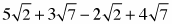2.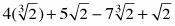3.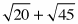4.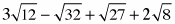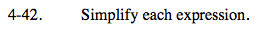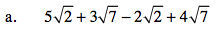$\text{Combine the }\sqrt{2}\text{'s with the }\sqrt{2}\text{s and the }\sqrt{7}\text{s}$

$\text{ with the }\sqrt{7}\text{s.}$

$3\sqrt{2}+7\sqrt{7}$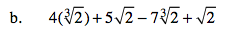$\sqrt{2}\text{ is different from }\sqrt{2}.$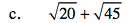Rewrite each term in simple radical form so that you can identify like terms.

$\sqrt{(4)(5)}+\sqrt{(9)(5)}$

$2\sqrt{5}+3\sqrt{5}$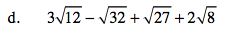$3\sqrt{4}\sqrt{3}-\sqrt{16}\sqrt{2}+\sqrt{9}\sqrt{3}+2\sqrt{4}\sqrt{2}$